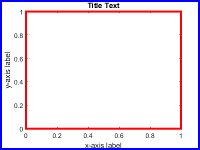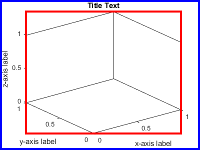# axes

## 语法

``axes``
``axes(Name,Value)``
``axes(parent,Name,Value)``
``ax = axes(___)``
``axes(cax)``

## 说明

``axes` 在当前图窗中创建默认的笛卡尔坐标区，并将其设置为当前坐标区。通常情况下，您不需要在绘图之前创建坐标区，因为如果不存在坐标区，图形函数会在绘图时自动创建坐标区。`

``axes(Name,Value)` 使用一个或多个名称-值对组参数修改坐标区的外观，或控制数据的显示方式。例如，`'FontSize',14` 可设置坐标区文本的字体大小。有关属性列表，请参阅 Axes 属性。`

``axes(parent,Name,Value)` 在由 `parent` 指定的图窗、面板或选项卡中创建坐标区，而不是在当前图窗中创建。`
``ax = axes(___)` 返回创建的 `Axes` 对象。可在创建 `Axes` 对象后使用 `ax` 查询和修改对象属性。有关属性列表，请参阅 Axes 属性。`
``axes(cax)` 将 `cax` 指定的坐标区或图设置为当前坐标区，并使父图窗成为焦点。此命令还将 `cax` 设置为图窗的 `Children` 属性中列出的第一个对象，并将图窗的 `CurrentAxes` 属性设置为 `cax`。`

## 示例

```figure ax1 = axes('Position',[0.1 0.1 0.7 0.7]); ax2 = axes('Position',[0.65 0.65 0.28 0.28]);``````contour(ax1,peaks(20)) surf(ax2,peaks(20))``````figure ax1 = axes('Position',[0.1 0.1 .6 .6],'Box','on'); ax2 = axes('Position',[.35 .35 .6 .6],'Box','on');````ax1` 设置为当前坐标区。此操作将使该坐标区显示在最前面，并使其成为后续图形函数的目标。在坐标区上添加一个线图。

```axes(ax1) x = linspace(0,10); y = sin(x); plot(x,y)``````figure tab1 = uitab('Title','Tab1'); ax1 = axes(tab1); plot(ax1,1:10) tab2 = uitab('Title','Tab2'); ax2 = axes(tab2); surf(ax2,peaks)```## 输入参数

```fig = gcf; fig.CurrentAxes = cax;```

### 名称-值对组参数

• `left``bottom` 元素定义从容器（通常为图窗、面板或选项卡）左下角到位置边界左下角之间的距离。

• `width``height` 元素是位置边界维度。对于三维视图中的坐标区，`Position` 属性是包围坐标区的最小矩形。• `left``bottom` 元素定义从容器（通常为图窗、面板或选项卡）左下角到外部位置边界左下角之间的距离。

• `width``height` 元素是外边界尺寸。`Units`说明
`'normalized'`（默认值）根据容器进行归一化，容器通常是图窗或面板。容器的左下角映射到 `(0,0)`，右上角映射到 `(1,1)`
`'inches'`英寸。
`'centimeters'`厘米。
`'characters'`

• 字符宽度 = 字母 `x` 的宽度。

• 字符高度 = 两个文本行的基线之间的距离。

`'points'`字体磅数。一磅等于 1/72 英寸。
`'pixels'`

• 在 Windows 系统上，一个像素是 1/96 英寸。

• Macintosh 系统上，一个像素是 1/72 英寸。

• 在 Linux® 系统上，一个像素的大小由系统分辨率确定。